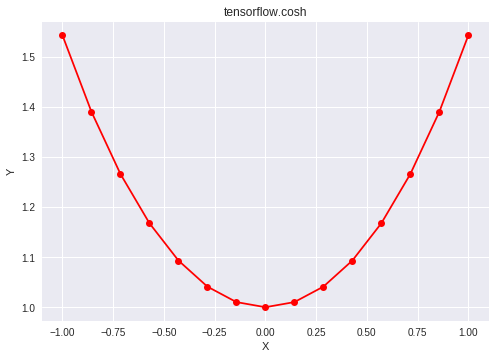# Python | Tensorflow cosh() method

Tensorflow is an open-source machine learning library developed by Google. One of its applications is to develop deep neural networks.
The module tensorflow.math provides support for many basic mathematical operations. Function tf.cosh() [alias tf.math.cosh] provides support for the hyperbolic cosine function in Tensorflow. It expects the input in radian form. The input type is tensor and if the input contains more than one element, element-wise hyperbolic cosine is computed.

Syntax: tf.cosh(x, name=None) or tf.math.cosh(x, name=None)
Parameters
x: A tensor of any of the following types: float16, float32, float64, complex64, or complex128.
name (optional): The name for the operation.
Return type: A tensor with the same type as that of x.

Code #1:

## Python3

 `# Importing the Tensorflow library` `import` `tensorflow as tf`   `# A constant vector of size 6` `a ``=` `tf.constant([``1.0``, ``-``0.5``, ``3.4``, ``-``2.1``, ``0.0``, ``-``6.5``],` `                               ``dtype ``=` `tf.float32)`   `# Applying the cosh function and` `# storing the result in 'b'` `b ``=` `tf.cosh(a, name ``=``'cosh'``)`   `# Initiating a Tensorflow session` `with tf.Session() as sess:` `    ``print``(``'Input type:'``, a)` `    ``print``(``'Input:'``, sess.run(a))` `    ``print``(``'Return type:'``, b)` `    ``print``(``'Output:'``, sess.run(b))`

Output:

```Input type: Tensor("Const_2:0", shape=(6, ), dtype=float32)
Input: [ 1.  -0.5  3.4 -2.1  0.  -6.5]
Return type: Tensor("cosh_1:0", shape=(6, ), dtype=float32)
Output: [  1.5430806   1.127626   14.998738    4.144313    1.        332.5716   ]```

Code #2: Visualization

## Python3

 `# Importing the Tensorflow library` `import` `tensorflow as tf`   `# Importing the NumPy library` `import` `numpy as np`   `# Importing the matplotlib.pyplot function` `import` `matplotlib.pyplot as plt`   `# A vector of size 15 with values from -1 to 1` `a ``=` `np.linspace(``-``1``, ``1``, ``15``)`   `# Applying the hyperbolic cosine function and` `# storing the result in 'b'` `b ``=` `tf.cosh(a, name ``=``'cosh'``)`   `# Initiating a Tensorflow session` `with tf.Session() as sess:` `    ``print``(``'Input:'``, a)` `    ``print``(``'Output:'``, sess.run(b))` `    ``plt.plot(a, sess.run(b), color ``=` `'red'``, marker ``=` `"o"``) ` `    ``plt.title(``"tensorflow.cosh"``) ` `    ``plt.xlabel(``"X"``) ` `    ``plt.ylabel(``"Y"``) `   `    ``plt.show()`

Output:

```Input: [-1.         -0.85714286 -0.71428571 -0.57142857 -0.42857143 -0.28571429
-0.14285714  0.          0.14285714  0.28571429  0.42857143  0.57142857
0.71428571  0.85714286  1.        ]
Output: [1.54308063 1.39039564 1.26613436 1.16775654 1.09325103 1.04109475
1.01022145 1.         1.01022145 1.04109475 1.09325103 1.16775654
1.26613436 1.39039564 1.54308063]```Whether you're preparing for your first job interview or aiming to upskill in this ever-evolving tech landscape, GeeksforGeeks Courses are your key to success. We provide top-quality content at affordable prices, all geared towards accelerating your growth in a time-bound manner. Join the millions we've already empowered, and we're here to do the same for you. Don't miss out - check it out now!

Previous
Next